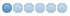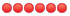# Some questions abput MRCC

• jamcas
• Topic Author
• Offline
• New Member
•5 months 1 day ago - 5 months 1 day ago #791 by jamcas
Hi, I'm a beginner for mrcc. There are some questions that puzzle me:

1.As we know, the ccsd(t) calculations in molpro can only be based on the RHF/ROHF reference, so i'm very intersted in mrcc which ccsd(t) calculations can be base on arbitrary single-reference. I tried to calculate a system in mrcc where the T1 value in molpro is a bit large in order to see the performance of using UHF as a reference. However, I get an abnormal result with a huge norm value. Is there any way such as the cc shift in molpro to improve the ccsd convergence? The molpro and mrcc outpu are attached.

2.Is there any way to print the T1 diagnostic value? It's vey useful to check the reliability of CCSD results.

3.Can mrcc handle the systems with multi-configurational character, such as open-shell singlet, based on the UHF reference?

Look forward to hearing from you soon, if you have any questions, please feel free to ask.
##### Attachments:
Last edit: 5 months 1 day ago by jamcas.

• kallay
• Offline
•• Mihaly Kallay
5 months 1 day ago #792 by kallay
Replied by kallay on topic Some questions abput MRCC
1) Thank you for pointing out this. Our four-center integral transformation routine does not function correctly if KS orbitals and the uccsd code are used. We should fix this. At the moment, you can use density fitting CCSD(T), that is, set calc=DF-CCSD(T) in the input.
2) With the public version of the uccsd code, it is not possible. We have already implemented it in the development version, which will be released soon.
3) In principle, it is possible, if you can get an UHF solution. Then you can run an MRCCSD calculation by setting calc=CCSD and nacto/nactv or active keyword as appropriate.

Best regards,
Mihaly Kallay

• jamcas
• Topic Author
• Offline
• New Member
•4 months 4 weeks ago #793 by jamcas
Replied by jamcas on topic Some questions abput MRCC
Thanks for your reply! When I set calc=DF-CCSD(T) the RI basis must be used and the DFT calculation is hard to convergence even set qscf=AugHessG. Here is my input:
basis=aug-cc-pVTZ
calc=DF-ccsd(t)
scftype=uhf
dft=user
2
1.0 b88
1.0 pw91c
mem=60GB
dfbasiscor=none
dfbasis_scf=none
agrid=LD0110-LD0974
ccmaxit=50000
scfmaxit=20000
mult=8
charge=-1
verbosity=3
qscf=AugHessG
unit=angs

geom=xyz
5

V                 -0.37577200   -0.61692500    0.00001200
V                 -2.06758100    0.02363600   -0.00001000
C                 -0.58122200    1.38919100    0.00000200
C                  0.72022300    1.21999200    0.00002000
Cr                 2.30679700   -0.08372700   -0.00000800

• kallay
• Offline
•• Mihaly Kallay
4 months 4 weeks ago #794 by kallay
Replied by kallay on topic Some questions abput MRCC
First, run an SCF calculation with the aug-cc-pVDZ basis:

basis=aug-cc-pVDZ
dfbasis_scf=def2-QZVPP-RI-JK
calc=SCF
dft=user
2
1.0 b88
1.0 pw91c
mem=60GB
mult=8
charge=-1
verbosity=3

Then restart the calculation from the aug-cc-pVDZ density:

basis=aug-cc-pVTZ
dfbasis_cor=def2-QZVPP-RI-JK
dfbasis_scf=def2-QZVPP-RI-JK
scfiguess=restart
calc=DF-CCSD(T)
dft=user
2
1.0 b88
1.0 pw91c
mem=60GB
mult=8
charge=-1
verbosity=3

(For the correlation fitting basis, you should look for other one as def2-QZVPP-RI-JK is probably not optimal for correlation fitting.)

Best regards,
Mihaly Kallay

• jamcas
• Topic Author
• Offline
• New Member
•4 months 4 weeks ago - 4 months 4 weeks ago #795 by jamcas
Replied by jamcas on topic Some questions abput MRCC
That's really useful for me to imporve the convergence of SCF! However the norm value in uccsd is still huge, so I try to use multireference ccsd to see if it can be improved. But program is stopped with an error:
Executing goldstone...

Generation of MRCC equations in terms of H and T...
Generation of antisymmetrized Goldstone diagrams...
Number of diagrams in T^1 equations:    15
Number of diagrams in T^2 equations:    38
Number of diagrams in T^3 equations:    54
Number of diagrams in T^4 equations:    81
Number of diagrams in T^5 equations:   106
Number of diagrams in T^6 equations:   142
Number of diagrams in T^7 equations:   176
Number of diagrams in T^8 equations:   222
Number of diagrams in T^9 equations:   266
Number of diagrams in T^10 equations:  323
Number of diagrams in T^11 equations:  378
Number of diagrams in T^12 equations:  447
Number of diagrams in T^13 equations:  514
Number of diagrams in T^14 equations:  596
Number of diagrams in T^15 equations:  676
Number of diagrams in T^16 equations:  772
Number of diagrams in T^17 equations:  861
Number of diagrams in T^18 equations:  285
Translation of diagrams to factorized equations...
Number of restricted diagrams in T^1 equations:    440
Number of restricted diagrams in T^2 equations:   3296
Unable to factorize.

Fatal error in exec goldstone.
Program will stop.
Here is my input:
basis=aug-cc-pvdz
calc=ccsd(t)
scftype=uhf
dft=user
2
1.0 b88
1.0 pw91c
mem=60GB
dfbasiscor=none
dfbasis_scf=none
agrid=LD0110-LD0974
ccmaxit=50000
scfmaxit=20000
mult=8
charge=-1
verbosity=3
active=serialno
6-20
scfiguess=restart
ccprog=mrcc

unit=angs
geom=xyz
5

V                 -0.37577200   -0.61692500    0.00001200
V                 -2.06758100    0.02363600   -0.00001000
C                 -0.58122200    1.38919100    0.00000200
C                  0.72022300    1.21999200    0.00002000
Cr                 2.30679700   -0.08372700   -0.00000800
Last edit: 4 months 4 weeks ago by jamcas.

• kallay
• Offline
•